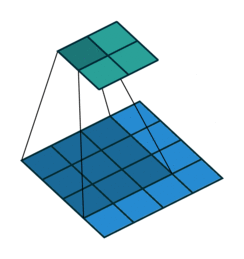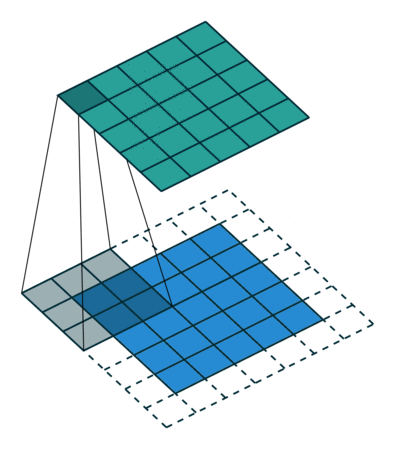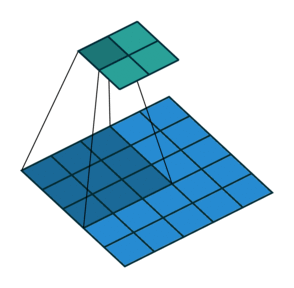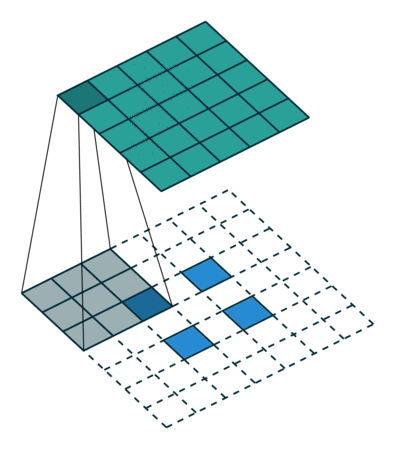Convolutional Autoencoder

By Prof. Seungchul Lee
http://iai.postech.ac.kr/
Industrial AI Lab at POSTECH

# 1. 2D Convolution¶

tf.nn.conv2d(input, filter, strides, padding)

input = tensor of shape [None, input_h, input_w, input_ch]
filter = tensor of shape [k_h, k_w, input_ch, output_ch]
strides = [1, s_h, s_w, 1]


• filter size

• the field of view of the convolution.
• stride

• the step size of the kernel when traversing the image.

• how the border of a sample is handled.
• A padded convolution will keep the spatial output dimensions equal to the input, whereas unpadded convolutions will crop away some of the borders if the kernel is larger than 1.
• 'SAME' : enable zero padding
• 'VALID' : disable zero padding
• input and output channels
• A convolutional layer takes a certain number of input channels ($C$) and calculates a specific number of output channels ($D$).

Examples

input = [None, 4, 4, 1]
filter size = [3, 3, 1, 1]
strides = [1, 1, 1, 1]input = [None, 5, 5, 1]
filter size = [3, 3, 1, 1]
strides = [1, 1, 1, 1]# 2. Transposed Convolution¶

• The need for transposed convolutions generally arises from the desire to use a transformation going in the opposite direction of a normal convolution, i.e., from something that has the shape of the output of some convolution to something that has the shape of its input while maintaining a connectivity pattern that is compatible with said convolution. For instance, one might use such a transformation as the decoding layer of a convolutional autoencoder or to project feature maps to a higher-dimensional space.
• Some sources use the name deconvolution, which is inappropriate because it’s not a deconvolution. To make things worse deconvolutions do exists, but they’re not common in the field of deep learning.

• An actual deconvolution reverts the process of a convolution.

• Imagine inputting an image into a single convolutional layer. Now take the output, throw it into a black box and out comes your original image again. This black box does a deconvolution. It is the mathematical inverse of what a convolutional layer does.

• A transposed convolution is somewhat similar because it produces the same spatial resolution a hypothetical deconvolutional layer would. However, the actual mathematical operation that’s being performed on the values is different.

• A transposed convolutional layer carries out a regular convolution but reverts its spatial transformation.

tf.nn.conv2d_transpose(value, filter, output_shape, strides, padding = 'SAME')

value = 4D tensor of shape [batch, input_h, input_w, input_ch]
filter = tensor of shape [k_h, k_w, output_ch, input_ch]
output_shape = [batch, output_h, output_w, output_ch]
strides = stride of the sliding window for each dimension of the input tensor


• 'SAME' : enable zero padding
• 'VALID' : disable zero padding

An image of 5x5 is fed into a convolutional layer. The stride is set to 2, the padding is deactivated and the kernel is 3x3. This results in a 2x2 image.2D convolution with no padding, no stride and kernel of 3

If we wanted to reverse this process, we’d need the inverse mathematical operation so that 9 values are generated from each pixel we input. Afterward, we traverse the output image with a stride of 2. This would be a deconvolution.

A transposed convolution does not do that. The only thing in common is it guarantees that the output will be a 5x5 image as well, while still performing a normal convolution operation. To achieve this, we need to perform some fancy padding on the input.Transposed 2D convolution with no padding, stride of 2 and kernel of 3

It merely reconstructs the spatial resolution from before and performs a convolution. This may not be the mathematical inverse, but for Encoder-Decoder architectures, it’s still very helpful. This way we can combine the upscaling of an image with a convolution, instead of doing two separate processes.

• Another example of transposed convolution